Published: 10 October 2014

# Electroacoustic methods of determining the parameters of speech sound generator

Maciej Kłaczyński1
Wiesław Wszołek2
1, 2AGH University of Science and Technology, Department of Mechanics and Vibroacoustics, Krakow, Poland
Corresponding Author:
Maciej Kłaczyński
Views 78

#### Abstract

The spectrum character of speech wave is connected with the fundamental frequency (F0) of human vocal folds vibration. As it is considered, F0 of the source during voicing contains an abundance of information on the larynx pathology, individual trait, the emotional state and ethnographic origin of speaker. The present paper presents results of research that conducted simultaneous measurement of fundamental frequency of vocal fold vibration by the electroglottography (EGG) and with the acoustic methods. The analysis of the ${F}_{0}$ function exactitude and the usefulness of these methods were executed too.

## 1. Introduction

The emitted speech signal is a source of useful diagnostic and prognostic information. Besides of the individual features of a speaker, the speech signal carries semantic and emotional state information, and other kinds, enabling to determine speaker’s ethnic origin, social status, education, and overall health. The speech signal can become, through selected parameters, an additional source of information on anatomic, physiological and pathological (deformation) conditions of human internal organs. A number of authors’ research proves that maximum information on phonetic action can be assembled by delimitation the parameters of speech sound generator such as the fundamental frequency ${F}_{0}$, short and long term frequency perturbations, short and long term amplitude perturbations, noise related, tremor, voice break and subharmonic. The fundamental tone function ${F}_{0}$ can be estimated by internal measurements (e.g. optical methods) or external measurements (like acoustic or electrical methods) [1, 2]. The optical methods include: stroboscopy, cinematography, videokymography (VKG), photoglottography (PGG), electrolaryngography (ELG) and two-point holographic interferometry. The acoustic methods include ultrasonography (USG), multi-dimension speech signal analysis and test evaluation of the voice acoustic pressure, while the electrical method is usually electroglottography (EGG). The literature demonstrates non numerous researches for Polish speech have conducted simultaneous measurement of fundamental frequency by the EGG and with the acoustic methods. The present paper presents results of such research. In this paper had been carried out the analysis of the accuracy of algorithms (zero crossing measure ZCM, cepstral analysis – CEPA, higher-order spectra analysis – HOSA) to determining the parameters of ${F}_{0}$, Jitter, Shimmer [3-7].

## 2. Speech signal production

An acoustic speech signal, defined as a variation of acoustic pressure in time, has a complex graph, being a reflection of its complex articulation process. On the parameters of the signal influence both its source (i.e. the vibrating vocal folds or sound caused by turbulent air flow through the narrowing of speech organs) and dynamical properties of the vocal channel, forming the structure of the signal. In the time domain the speech signal $p\left(t\right)$ can be mathematically described using a convolution of time-dependent signal source $g\left(t\right)$ and pulselike answer of the voice channel $h\left(t\right)$ :

1
$p\left(t\right)={\int }_{0}^{t}h\left(t-\tau \right)g\left(\tau \right)d\tau .$

Interpretation of Eq. (1) indicates that in the time-dependent acoustic speech signal the properties of the source and the properties of the sound forming voice channel are closely related (Fig. 1).

Fig. 1a) Acoustic speech signal, b) vibrations of vocal folds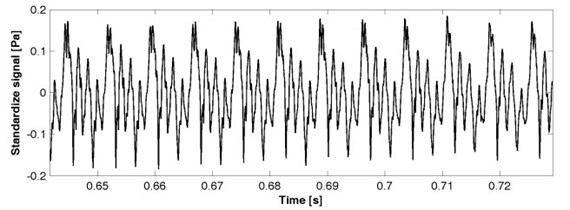a)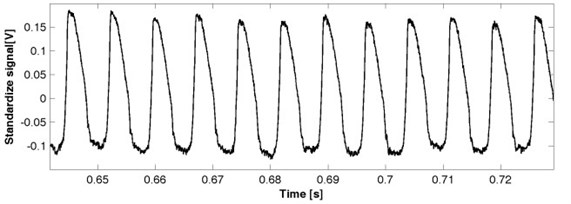b)

The repetition time (period) of the vocal cords vibrations is called fundamental frequency ${F}_{0}$ and approximate value can be expressed by the following formula :

2
${F}_{0}=\frac{1}{2\pi }\sqrt{\frac{s}{m}},$

where $m$ – mass of the vibrating vocal [kg], $s$ – stiffness constant of the chords [N/m].

The fluctuation of the fundamental frequency and signal amplitude can be estimate by Jitter and Shimmer parameters. Jitter ($Jitt$) denotes the deviation of the larynx tone frequency in consecutive cycles from the average frequency of the larynx tone according formula:

3
$Jiit=\frac{\frac{1}{N-1}{\sum }_{i=1}^{N-1}\left|{F}_{0}^{\left(i\right)}-{F}_{0}^{\left(i+1\right)}\right|}{\frac{1}{N}{\sum }_{i=1}^{N-1}{F}_{0}^{\left(i\right)}}\bullet 100%,$

where $N$ – number of instantaneous signal periods.

Shimmer ($Shim$) denotes the deviation of the larynx tone amplitude in the consecutive cycles from the average amplitude of the larynx tone according formula:

4
$Shim=\frac{\frac{1}{N-1}{\sum }_{i=1}^{N-1}\left|{A}_{0}^{\left(i\right)}-{A}_{0}^{\left(i+1\right)}\right|}{\frac{1}{N}{\sum }_{i=1}^{N-1}{A}_{0}^{\left(i\right)}}\bullet 100%,$

where ${A}_{0}$ – amplitude of fundamental frequency in instantaneous signal periods.

## 3. Research material and methodology

The goal of this research and analysis was to determine the difference between the ${F}_{0}$, Jitter and Shimmer estimation from the acoustic signals and the EGG signals during phonation. The experiment was carried out on the group of 328 people, both men and women, age 19 to 80 years, so-called standards of Polish language, without any pathologies that could affect the voice quality.

The time-dependent acoustic speech signal and the EGG signal were recorded simultaneously in an anechoic chamber, at the Department of Mechanics and Vibroacoustic, AGH University of Science and Technology, Kraków, Poland. The diagram of the measurement setup is shown in Fig. 2.

Fig. 2The block diagram of the measuring setup, where: 40 AF – G.R.A.S microphone, 1201 – Norsonic preamplifire, 12AA – G.R.A.S amplifire, 1314 – M-AUDIO IN/OUT chart, 6103 – Kayelemetrics Electroglottograph (EGG)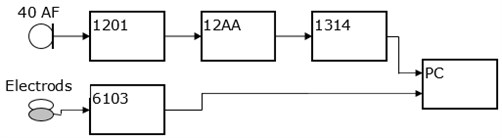The task of the group people being the subjects of examination was to read out the phonetic text slowly and without any intonation. They had to repeat three times: the vowels – a, e, i, u; the vowels with the prolonged phonation – a, e, i, u; the words – “ala”, “as”, “ula”, “ela”, “igła” (i.e. Polish names and Polish equivalent for “needle”) and the sentence – “dziś jest ładna pogoda” (i.e. the Polish equivalent of the sentence “We have a good weather today”).

## 4. Results

To depict and compare the ${F}_{0}$, determined by the acoustic and the electroglotographic methods, the analysis in the frequency domain was made, using Short Term Fourier Transform (STFT). Before frequency analysis, the data were subjected to the process of preemphasis with the band-pass FIR filter, with ${f}_{low}=$50 Hz and ${f}_{upp}=$ 400 Hz. The dynamical spectrum $W\left(t,f\right)$ containing 56 lines with the $f=$10 Hz width, made with the $t=$0.1 s time quantum and the level quantum equal to $L=$0.2 dB, was obtained. The subject of analysis was 4 vowels pronounced by each person (3936 records – 328 persons × 4 vowels × 3 expression). The goal of the analysis was to determine the difference between the spectra obtained from the acoustic and the EGG signals. These vowels have a fundamental significance in the examination of the voice channel condition (especially of the glottis) because of their stationary-like time dependence. The examples of the over-time-averaged spectra of the vowels with the prolonged phonation, obtained from the EGG and the acoustic signals, are presented in Fig. 3.

Analysis of the frequency spectra carried out for each investigated signal sample, showed only minor differences (in shape and envelope) between the fundamental tone spectra determined from the acoustic signal and from the EGG signals. The substantial differences, observed in the relative level (amplitude) of recorded signal, are related to the signal normalization process. For each group (acoustic signal sample, EGG signal sample), the averaged minimal value for all samples recorded in the given group was used as a reference level in the logarithmic scale.

In the second part of this research, comparison between the averaged values of ${F}_{0}$ obtained by the acoustic methods and the ${F}_{0}$ value determined with the help of EGG was made. The algorithms carrying out the detection of ${F}_{0}$ based on the zero crossing measure, higher-order spectra analysis, cepstral analysis were also implemented in the MATLAB environment. Estimation of relative error and standard uncertainty were done. Table 1 details a sample results for ${F}_{0}$ determined in acoustic methods for the /a/ vowel and these are displayed “vis a vis” results for ${F}_{0}^{*}$, determined by the EGG method. Table 2 shows an example results of Jitter and Shimmer parameters estimation.

Fig. 3Averaged spectrum of the vowel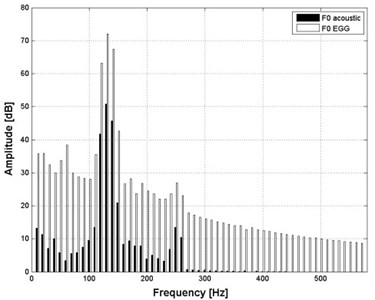a) “a” with the prolonged phonation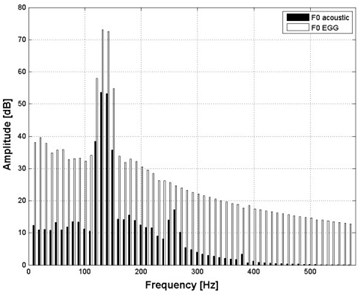b) “i“ with the prolonged phonation

Table 1Example results for calculation of relative error of F0 function for the “e” vowel with prolonged phonation

 ID of sample ${F}_{0}^{*}$ [Hz] (EGG) ${F}_{0}$ [Hz] (ZCM) ${F}_{0}$ [Hz] (CEPA) ${F}_{0}$ [Hz] (HOSA) $\mathrm{\Delta }{F}_{0}$ % (ZCM) $\mathrm{\Delta }{F}_{0}$ % (CEPA) $\mathrm{\Delta }{F}_{0}$ % (HOSA) 1 119 119 119 119 0 0 0 2 109 109 109 109 0 1 0 3 101 101 107 100 0 6 1 4 98 99 104 98 1 6 0 5 126 128 122 126 1 3 0 6 123 122 121 123 0 2 0 7 108 108 108 108 0 0 0 8 123 123 121 123 0 2 0 9 94 94 101 94 0 8 0 10 120 120 118 119 0 1 0

Table 2Example results of Jitter and Shimmer estimation

 Jitter [%] Shimmer [%] ZCM 0.02 0.03 CEPA 0.01 0.08 HOSA 0.02 0.09 EGG 0.02 0.02

## 5. Conclusions

The data analysis showed that for all analyzed vowels (the prolonged phonation), the mean squared error for the determination of ${F}_{0}$ by using the acoustic methods does not exceed 2 Hz for the zero crossing measure (ZCM), 1.5 Hz for the cepstrum algorithm (CEPA), and only 1 Hz for the higher-order spectra analysis (HOSA). This makes clear that the acoustic methods for ${F}_{0}$ derivation are effective and accurate, and can be treated as precise tools for the examination of non-pathologic ${F}_{0}$ derived from a healthy glottis.